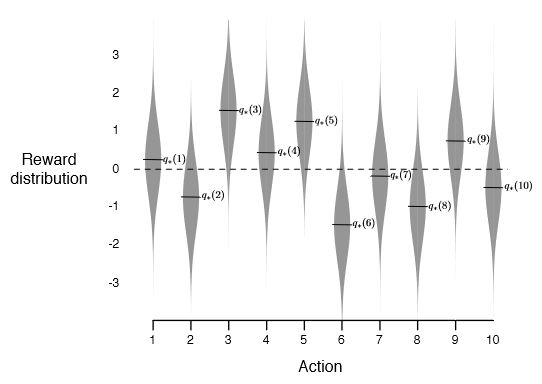# Chapter 2 Multi-armed Bandits

## Part I: Tabular Solution Methods

Tabular: the state and action spaces are small enough for the approximate value function to be presented as arrays, or tables.

In this ways, the methods can often find exact solutions.

Bandit problems: there is only a single state.

General problem formation: finite Markov decision proccesses. Including Bellman equations and value functions.

Three fundamental classes of methods for solving finite Markov decision processes: dynamic programming, Monte Carlo methods, and temporal-difference learning. And will introduce their combination.

Reinforcement learning uses evaluative feedback.

Purely evaluative feedback: how good the action taken was.

Purely instructive feedback: indicates the correct action to take, independent of the action really taken.

We will explore nonassociative settings (brandit) first.

## 2.1 A $k$-armed Bandit Problem

$k$-armed brandit: maximize total reward over some time period by choosing among $k$ options, and the value of action $a$ at time t, is denoted by:

$$q_*(a) = \mathbb{E}[R_t|A_t=a]$$

and we have $Q_t(a)$, which is our estimated value for $q_*(a)$ at time step $t$.

Greedy action: the action at time $t$ with the greatest estimated value.

Exploitation and exploration, the conflict between them.

## 2.2 Action-value Methods

Action-value methods: A set of methods to estimate the value for each action.

Sample range method: use the sample mean to estimate

$$Q_t(a)= \frac{\text{sum of rewards when }a\text{ taken prior to }t} {\text{number of times }a\text{ taken prior to }}=\frac{\sum^{t-1}_{i=1}R_i \cdot \mathbb{1}_{A_i=a}}{\sum^{t-1}_{i=1}{\mathbb{1}_{A_i=a}}}$$

Greedy action:

$$A_t\doteq \argmax_a Q_t(a)$$

$\varepsilon$-greedy methods: take greedy action most of the time, but randomly select all the actions with a probability of $\varepsilon$.

## 2.3 The 10-armed Testbed

10-armed testbed: a suite of test tasks, where we test the problem and record the rewards.• If $\varepsilon=0$, there is a chance that the greedy method will stuck performing suboptimal actions.
• If $\varepsilon\neq0$, then the larger $\varepsilon$ is, the quicker it will reach to its optimal action, but the highest average reward will be lower in the end.

However, if the reward variance is $0$, then greedy method will perform better than $\varepsilon$-greedy methods.

But if the bandit task is nonstationary, exploration is needed even in the deterministic case (where variance is $0$).

## 2.4 Incremental Implementation

Basic idea: it is not efficient (time and space efficient) to compute the average of all rewards $R$. So, we have $$Q_{n+1}=Q_n+\frac1n[R_n-Q_n]$$ or, in my own understanding, $$Q_{n+1}=\frac{n-1}{n}\cdot Q_n+\frac1n R_n$$ This rule is of form $$NewEstimate\leftarrow OldEstimate+StepSize[Targent-OldEstimate]$$ and the expression $[Targent-OldEstimate]$ is an error in the estimate.

## 2.5 Tracking a Nonstationary Problem

Basic idea: if the award is nonstationary, we would like to give more weight to the reward of more recent action, se we have

$$Q_{n+1}=Q_n+\alpha[R_n-Q_n],\ \alpha\in(0,1]$$

which results in

$$Q_{n+1}=(1-\alpha)^nQ_1+\sum^n_{i=1}\alpha(1-\alpha)^{n-i}R_i$$

and this is called exponential-recency-weighted average.

To assure convergence with probability $1$, we need to ensure that

$$\sum_{n=1}^\infty a_n(a)=\infty\text{ and }\sum_{n=1}^\infty a^2_n(a)<\infty$$ The first condition guarantees that the steps are large enough to eventually overcome any initial conditions or fluctuations. The second condit8on guarantees that eventually the steps become small enough to ensure convergence.

Note, convergence is not necessary, if $\alpha$ is a constant, the second condition is not met, thus it will not reach convergence but vary in response to the recently received rewards. And this is desierable in non-stationary results.

## 2.6 Optimistic Initial Values

Initial action values can also be used as a simple way to encourage exploration, the method of this is called optimistic initial values.

## 2.7 Upper-Confidence-Bound Action Selection

Basic idea: select among the non-greedy actions according to their potential for actually being optimal.

One effective way of doing so is to select actions according to upper-confidence-bound action selection (UCB):

$$A_t\doteq\argmax_a\left[Q_t(a)+c\sqrt{\frac{\ln{t}}{N_t(a)}}\right]$$

Idea of UCB:

1. square-root term: a measure of the uncertainty or variance in the estimate of $a$'s value.
2. The quantity being max'ed over is a sort of upper bound on the possible true value of action $a$.
3. $c$ determines the confidence level.

UCB often performs well but more difficult to generalize to more RL settings.

Basic idea: insdead of estimate the value, we can learn a numerical preference for each action, denoted by $H_t(a)$.

We determine actions probabilities according to a soft-max distribution

$$\Pr{A_t=a}\doteq\frac{e^{H_t(a)}}{\sum_{b=1}^{k}{e^{H_t(b)}}}\doteq\pi_t(a)$$

### A Natural Learning Algorithm

After selecting action $A_t$ and receiving reward $R_t$

$$H_{t+1}(A_t)\doteq H_t(A_t)+\alpha(R_t-\bar{R_t})(1-\pi_t(A_t))\text{, and}$$ $$H_{t+1}(a)\doteq H_t(a)-\alpha(R_t-\bar{R_t})\pi_t(a)\text{ for all }a\neq A_t$$

One benifit of this is its adaptation to changeable value.

## 2.9 Associative Search (Contextual Bandits)

Associative tasks: tasks in which we need to associate different actions with different situations.

Associative tasks are intermediate between the $k$-armed bandit problem and the full reinforcement learning problem. If actions are allow to affect the next situation as well as the reward, the this is a full reinforcement learning problem.

Below are sections from book Bandit Algorithms, chapter 4, Stochastic Bandits

## 4.2 The Learning Objective

Let $X$ to be the reward, the learning objective is to maximize the total reward $S_n=\sum^n_{t=1}X_t$.

## 4.4 The Regret

Regret: the deficit suffered by the learner relative to the optimal policy.

Let $\nu=(P_a:a\in \mathcal{A})$ be a stochastic bandit and define

$$\mu_a(\nu)=\int^\infty_{-\infty}{x\ dP_a(x)}$$

Then let $\mu^*(\nu)=\max_{a\in \mathcal{A}}\mu_a(\nu)$ be the largest mean of all the arms.

The regret of policy $\pi$ on bandit instance $\nu$ is

$$R_n(\pi,\mu)=n\mu^*(v) - \mathbb{E}\left[ \sum^n_{t=1}X_t \right]$$

Lemma. Let $\nu$ be a stochastic bandit environment

1. $R_n(\pi,\nu)\geq 0$ for all policies $\pi$
2. The policy $\pi$ choosing $A_t\in\argmax_a\mu_a$ for all $t$ satisfies $R_n(\pi,\mu)=0$
3. if $R_n(\pi,\mu)=0$ for some policy $\pi$, then $\mathbb{P} (\mu_{A_{t}}=\mu^*)=1$ for all $t \in [n]$

A relatively week objective $$\text{for all }\mu\in\mathcal{E}\text{, }\lim_{n\rightarrow\infty}{\frac{R_n(\pi,\nu)}{n}}=0$$

Yet another alternative is to find a function $C: \mathcal{E}\rightarrow[0,\infty)$ and $f:\mathbb{N}\rightarrow[0,\infty)$ such that

$$\text{for all }n\in\mathbb{N}\text{, }\nu\in\mathcal{E}\text{, }R_n(\pi,\nu)\leq C(\nu)f(n)$$

Bayesian regret $$\text{BR}_n(\pi,Q)=\int_{\mathcal{E}}R_n(\pi,\nu)dQ(v)$$